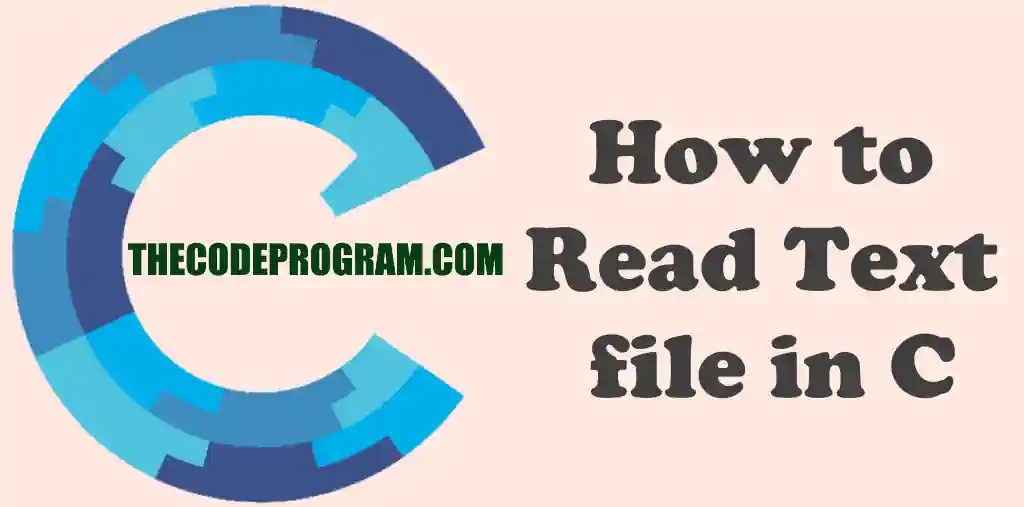## How to Read Text file in C

Hello everyone, in this article we are going to see how can we read a file until the end and assign line by line to a variaable in C programming language with an example.

Let's get started.

First we are going to need below libraries. So include them:
``````
#include <stdio.h>
#include<stdlib.h>
``````
Now rest of codes are will be in int main( void ) { ... } function.
First we request from user to specify the file
``````
char filePath;
printf( "Enter file or drag file here to get file path :");
gets( filePath );
``````
Then we load inputted file path to the FILE structure to read
also we need a variable to read the text line by line
Also we can count how many lines do we have in text file
``````
char const* const fileName = filePath;
FILE* file = fopen(fileName, "r");

char line;

char c;
int lineCount = 0;
``````
Now we check the text file is correct and count the newlines
``````
for (c = getc(file); c != EOF; c = getc(file))
if (c == '\n')
lineCount++;
``````
Here we clear the related pointer and reload the file
``````
fclose(file);
file = fopen(fileName, "r");
``````
Here you can make some controls on the characters EOF = end of file, You can make this control with different ways you can use ASCII encoding or whatever you want
``````
for (c = getc(file); c != EOF; c = getc(file)){
if (!(c == '0' || c == '1' || c == '2'   c == 'a' || c == 'b' || c == 'c' ||c == '\n')){
return 0;
}
}
``````
Again we clear the related pointer and reload the file.
``````
fclose(file);
file = fopen(fileName, "r");
``````
Lastly we print all text line by line and close the file when operation is completed
``````
int i = 0;
while (fgets(line, sizeof(line), file)) {
i++;
printf("Line %d : %s", i, line);
}
printf("\n");
fclose(file);
``````
I have commented the control section to show you that the example is working. Do not forget there when you are in need.

Burak Hamdi TUFAN.

04/09/2020

30/08/2021

06/04/2023